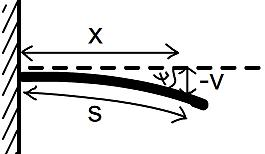#### You may also like### Overarch 2

Bricks are 20cm long and 10cm high. How high could an arch be built without mortar on a flat horizontal surface, to overhang by 1 metre? How big an overhang is it possible to make like this?### Stonehenge

Explain why, when moving heavy objects on rollers, the object moves twice as fast as the rollers. Try a similar experiment yourself.### Maximum Flow

Given the graph of a supply network and the maximum capacity for flow in each section find the maximum flow across the network.

# Beam Me Up

##### Age 16 to 18 Challenge Level:

The curvature, $\kappa$, of a circle of radius $r$ (given by the equation $y=\sqrt{r^2-x^2}$) is defined to be $\frac{1}{r}$. The curvature of a more general curve $y=f(x)$ varies from point to point, and equals the curvature of the circle which just matches the 'bend' of the curve at that point. It has the formula

$$\kappa = \frac{y''}{(1+y'^2)^{\frac{3}{2}}}\;.$$

The angle $\psi$ by which a point on a curve has deviated from a straight line is $\psi = \int{\kappa}ds$. i.e. the angle deviated is the sum of the curvature up to that point. Think about this formula. Does it make sense? Just think about curvature for circles to begin with.In structures, we generally expect the deflections of solid objects to be small. (Think of how little a bridge moves up and down when you walk on it, relative to its length.) So we can usually approximate the distance along a curved beam, $s$, as the linear distance, $x$, i.e. $\delta s \approx \delta x$.

Can you work out a formula for the vertical deflection (displacement) $v$ of a point on the curve, in terms of $\psi$ and $x$?

What about $v$ in terms of $\kappa$ and $x$?